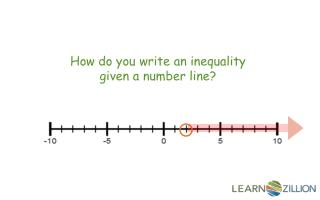DownloadDownload PresentationHow do you write an inequality given a number line ?

# How do you write an inequality given a number line ?

Download Presentation## How do you write an inequality given a number line ?

- - - - - - - - - - - - - - - - - - - - - - - - - - - E N D - - - - - - - - - - - - - - - - - - - - - - - - - - -
##### Presentation Transcript

1. How do you write an inequality given a number line?

2. In this lesson you will learn to write an inequality given a number line representation.

3. A variable is a letter or symbol that represents an unknown number or set of numbers. For example, x or ★ could be used to represent unknown numbers. An inequality has more than 1 solution.

4. represents: An open circle > greater than < less than represents: A closed circle ≥greater than or equal to ≤ less than or equal to

5. The highlighted numbers are part of the solution set of the inequality. x could equal 2.3, 5, 10, 13½, 30, 500…

6. What inequality represents the number line below?

7. 1. Identify circle location 2. Identify type of circle 3. Identify direction of shading

8. 1. -5 2. Open circle (> or <) 3. Numbers are greater than -5

9. In this lesson you have learned to write an inequality given a number line representation.

10. What inequality represents the number line below?

11. Given inequality representations, write a word problem that matches the inequality. • 1. x < -3 • 8 ≥ x • 3.

12. Each player has a white board and a stack of number line inequality cards face down. Flip the top card and the first player to write the inequality correct keeps the card. The player with the most cards wins.

13. What inequality represents the number line below? • x ≤ 1 • x ≤ -1 • x ≥ 1 • x <1

14. What inequality represents the number line below? • x > -9 • x ≥ -9 • x > -11 • x <-11